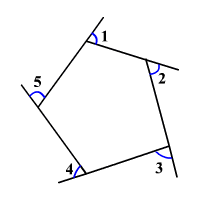# Polygon Exterior Angle Sum Theorem

If a polygon is convex, then the sum of the measures of the exterior angles, one at each vertex, is $360°$ .

Consider the sum of the measures of the exterior angles for an $n$ -gon.

The sum of the measures of the exterior angles is the difference between the sum of measures of the linear pairs and the sum of measures of the interior angles.

That is, the sum of the exterior angles $n$ is

$N=180n-180\left(n-2\right)$

Distribute $180$ .

$\begin{array}{l}N=180n-180n+360\\ \text{\hspace{0.17em}}\text{\hspace{0.17em}}\text{\hspace{0.17em}}\text{\hspace{0.17em}}\text{\hspace{0.17em}}\text{\hspace{0.17em}}=360\end{array}$

Example:$m\angle 1+m\angle 2+m\angle 3+m\angle 4+m\angle 5=360°$

(In the case of a non-convex polygon, you may need to consider some of the exterior angles as negative values.)